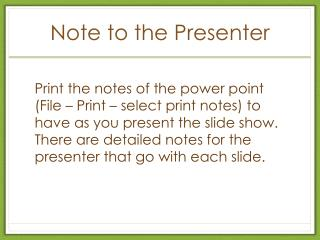# Note to the Presenter - PowerPoint PPT PresentationDownload PresentationNote to the Presenter

Note to the PresenterDownload Presentation## Note to the Presenter

- - - - - - - - - - - - - - - - - - - - - - - - - - - E N D - - - - - - - - - - - - - - - - - - - - - - - - - - -
##### Presentation Transcript

1. Note to the Presenter Print the notes of the power point (File – Print – select print notes) to have as you present the slide show. There are detailed notes for the presenter that go with each slide.

2. Investigating Properties of Real Numbers Commutative, Associative, Identity Properties of Addition and Multiplication Distributive Property of Multiplication over Addition Additive and Multiplicative Inverse Properties Multiplicative Property of Zero

3. Changes in the SOL • The properties are now taught in the following order:

4. Commutative Property of Addition 3+5 = 8 5+3 = 8 Does the order in which we add two quantities matter? That is, does a+b = b+a ? Let’s use Cuisinaire Rods to investigate the property. Is 3+5 the same as 5+3? They are the same because both have a length of 8 units.

5. Commutative Property of Multiplication Does the order in which we multiply two quantities matter? That is, does a x b = b x a ? Let’s use counters to investigate the property. Is 6x2 the same as 2x6? They are the same because both equal 12.

6. Commutative Property of Multiplication We can also use grid paper to investigate. Cut out a rectangle with 2 rows and 6 columns and another with 6 rows and 2 columns. They have the same area of 12 square units.

7. Associative Property of Addition Does the way in which we group quantities when adding matter? That is, does a+(b+c) = (a+b)+c ? Let’s use Cuisinaire Rods to investigate the property. Is 2+(3+5) the same as (2+3)+5? They are the same because both have a length of 10 units.

8. Associative Property of Multiplication Does the way in which we group quantities when multiplying matter? That is, does a(bc) = (ab)c ? Let’s use counters to investigate the property. Are 3x(2x6) and (3x2)x6 the same? They are both equivalent to 36.

9. Distributive Property of Multiplication over Addition Does the product of a number and a sum equal the sum of the individual products? That is, does a(b+c) = ab+ac ? Let’s use counters to investigate the property. Are 2(3+5) and 2x3+2x5 the same? They are both equivalent to 16.

10. Identity Properties For Addition and Multiplication Adding or Multiplying a number by an identity number retains the “identity” or original value of that number What number can we add to 5 and not change its value? Zero 0+5 = 5 and 5+0 = 5 What number can we multiply by 6 and not change its value? One 1x6 = 6 (one group of six) and 6x1 = 6 (six groups of one)

11. Multiplicative Property of Zero What happens when you multiply by zero? The result is zero. a x 0 = 0 and 0 x a = 0 Discuss how 0x6 (zero groups of six) and 6x0 (six groups of zero) both result in 0.

12. Inverse Property for Multiplication The inverse property of multiplication tells us that two numbers are inverses if their product is one (the multiplicative identity). That is, Let’s use pattern blocks to show and

13. Inverse Property for Multiplication Lay out 4 unit pieces. One-forth of four gives one unit piece.

14. Inverse Property for Multiplication Lay out three half pieces Two-thirds of three-halves gives two halves which is equivalent to one unit piece

15. Inverse Property for Multiplication 3 groups of what will equal 1? Make 3 groups Take a unit piece and divide it into three pieces. Put one piece in each group.

16. Discussion • What did you learn from this session? • How would you apply this to your classroom? • What is still unclear? • Comments and/or concerns?